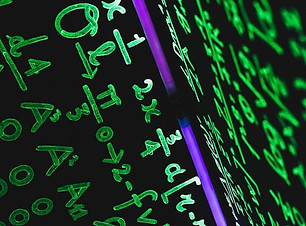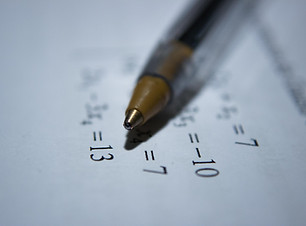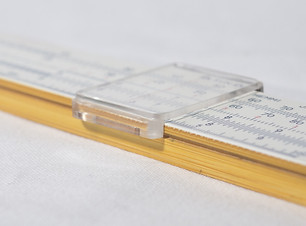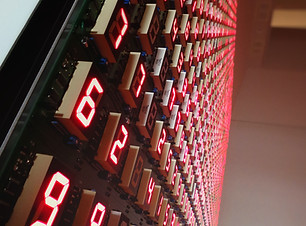# NCERT Solutions for Class 6- Free PDF for the academic year 2020-21

## NCERT Solutions for Class 6 Maths  for all the exercises from Chapters 1 to 14 are provided here.## Knowing Our Numbers

1.1 Introduction

1.2 Comparing Numbers

1.3 Large Numbers in Practice

1.4 Using Brackets

1.5 Roman Numerals## Basic Geometrical Ideas

4.1 Introduction

4.2 Points

4.3 A Line Segment

4.4 A line

4.5 Intersecting Lines

4.6 Parallel Lines

4.7 Ray

4.8 Curves

4.9 Polygons

4.10 Angles

4.11 Triangles

4.13 Circles## Fractions

7.1 Introduction

7.2 A Fraction

7.3 Fraction on the Number Line

7.4 Proper Fractions

7.5 Improper and Mixed Fractions

7.6 Equivalent Fractions

7.7 Simplest Form of a Fraction

7.8 Like Fractions

7.9 Comparing Fractions

7.10 Addition and Subtraction of Fractions## Mensuration

10.1 Introduction

10.2 Perimeter

10.3 Area## Symmetry

13.1 Introduction

13.2 Making Symmetric Figures : Ink-blot Devils

13.3 Figures With Two Lines of Symmetry

13.4 Figures with Multiple Lines of Symmetry

13.5 Reflection and Symmetry## Whole Numbers

2.1 Introduction

2.2 Whole Numbers

2.3 The Number Line

2.4 Properties Of Whole Numbers

2.5 Patterns in Whole Numbers## Understanding Elementary Shapes

5.1 Introduction

5.2 Measuring Line Segments

5.3 Angles-’Right’ and ‘Straight’

5.4 Angles- ‘Acute’, ‘Obtuse’ and ‘Reflex’

5.5 Measuring Angles

5.6 Perpendicular Lines

5.7 Classification of Triangles

5.9 Polygons

5.10 Three Dimensional Shapes## Decimals

8.1 Introduction

8.2 Tenths

8.3 Hundredths

8.4 Comparing Decimals

8.5 Using Decimals

8.6 Addition of Numbers with Decimals

8.7 Subtraction of Decimals## Algebra

11.1 Introduction

11.2 Matchstick Patterns

11.3 The Idea Of  A Variable

11.4 More Matchstick Patterns

11.5 More Examples of Variables

11.6 Use Of Variables in Common Rules

11.7 Expressions with Variables

11.8 Using Expressions Practically

11.9 What is an Equation?

11.10 Solution of an Equation## Practical Geometry

14.1 Introduction

14.2 The Circle

14.3 A Line Segment

14.4 Perpendiculars

14.5 Angles## Playing With Numbers

###### 3.1 Introduction 3.2 Factors and Multiples 3.3 Prime and Composite Numbers 3.4 Test For Divisibility Of Numbers 3.5 Common Factors and Common Multiples 3.6 Some More Divisibility Rules 3.7 Prime Factorisation 3.8 Highest Common Factor 3.9 Lowest Common Multiple 3.10 Some Problems on HCF and LCM## Integers

6.1 Introduction

6.2 Integers

6.4 Subtraction of Integers with the help of a Number Line## Data Handling

9.1 Introduction

9.2 Recording Data

9.3 Organisation of Data

9.4 Pictograph

9.5 Interpretation of a Pictograph

9.6 Drawing a Pictograph

9.7 A Bar Graph## Ratio and Proportion

12.1 Introduction

12.2 Ratio

12.3 Proportion

12.4 Unitary Method